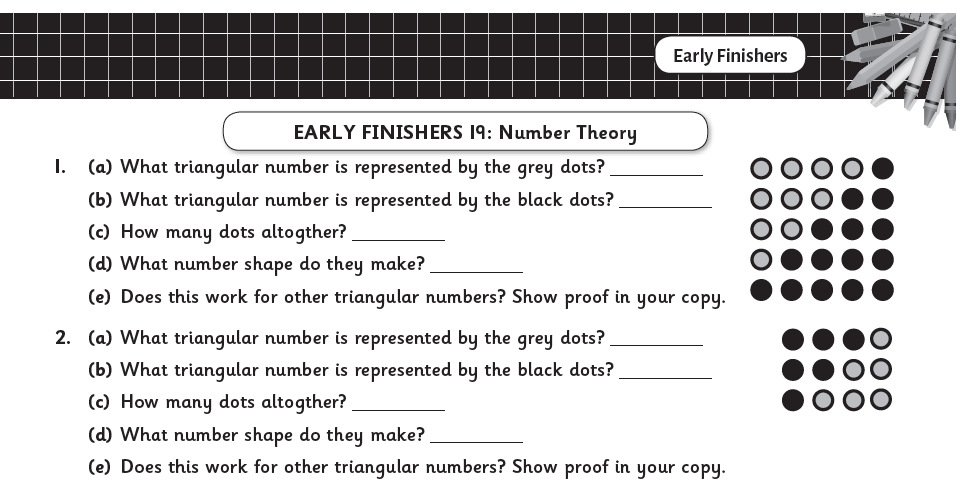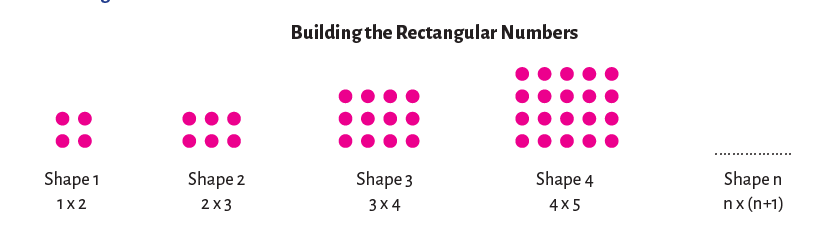# Digging Deeper into … Number Theory (5th & 6th classes)

## Digging Deeper into … Number Theory (5th & 6th classes)

For practical suggestions for families, and links to useful digital resources, to support children learning about the topic of number theory, please check out the following post: Dear Family, your Operation Maths Guide to Number Theory

Number Theory is a number topic that is concerned with subcategories of whole numbers: odd and even; factors and multiples; prime and composite; square, triangular and rectangular numbers; square roots and exponential numbers.

While 5th class is the first time that the children formally meet this strand unit, they have engaged with elements of the topic in previous classes:

• odd and even numbers were explored formally in 1st, 2nd and 3rd classes
• the language of factors and multiples was used in 3rd, 4th and 5th classes as part of the multiplication and division chapters.

### Concrete-pictorial-abstract approach (CPA)

Traditionally this topic is often taught in a quite abstract way e.g. using operations to calculate square numbers, square roots identifying prime numbers by the number of  factors, etc. However, number theory by its nature, prompts us to consider and explore the shape and arrangements of numbers and, thus, is ideal to be explored in a visual way. Even Lego can be used to explore square numbers, arrays and factors!

Therefore, the activities will once again follow a concrete-pictorial-abstract approach, as is used throughout Operation Maths with the emphasis being on the children being able to build and/or visualise the shape of the numbers themselves, before progressing to using more abstract means to identify larger numbers, compare patterns, etc.Therefore, the focus in this topic should not be on the numerals themselves, rather on the shape of the numbers; it is one thing to recognise or identify odd and even numbers, factors, prime, composite, squared numbers, cubed numbers, etc., it’s another thing to visualise them. Using concrete materials or pictorial representations is vital for the children to really develop their number sense and their appreciation of these numbers and how they relate to,  and interact with, each other.

### Number Theory in the real world

This topic is very interesting in the way it relates to spatial arrangements in real life. Triangular numbers can be seen in arrangements of bowling skittles, snooker balls, displays in the supermarket. Even numbers are evident wherever there is a pair.Encourage the children to suggest examples and applications of odd and even numbers, prime and composite numbers, squared numbers, cubed numbers, etc. in the world around them. The idea of videos going viral on the internet, rumours or secrets being spread can all be related back to exponential numbers.

And as the children explore more, they may also begin to discover other fascinating connections between various types of numbers such as the total of two consecutive triangular numbers is always a square number and that double any triangular number is always a rectangular number. Again the emphasis should be on the children discovering this for themselves through engagement in rich tasks, such as the one shown below from the Early Finishers photocopiable in the TRB.### Points to note for the teacher

• Counting/Natural numbers: When we talk about numbers in this topic we are referring to the set of counting numbers (i.e. 1, 2, 3, 4 … ) or natural numbers. These are whole numbers (no fractions or decimals) and do not include negative numbers. Since there is no universal agreement about whether to include zero in the set of natural numbers (some define the natural numbers to be the positive integers {1, 2, 3, …}, while for others the term designates the non-negative integers {0, 1, 2, 3, …}) in Operation Maths we only consider the positive integers, i.e. not including zero.
• That which constitutes a rectangular number is undefined in the mathematics curriculum and the definition varies from source to source, with many conflicting with each other, particularly online. In Operation Maths, we have gone with the definition, as shown in the image below, given in the NCCA Bridging Glossary. This defines rectangular numbers as n x (n+1) which can also be expressed as n² + n; thus, the 6th rectangular number is 6 x 7 or 6² + 6 both of which equal 42.• Only whole numbers can be even or odd. The children may think that 1.2 or 4.36 are even numbers since their last digits is one of 0, 2, 4, 6, 8. However, only whole numbers can be denoted even or odd; you cannot create either an even or odd cube pattern (as shown in the Operation Maths 5 Pupils’ Book, p. 153), using 1 and a bit cubes.
• Factors V multiplicands. In this sentence, 1.2 × 1.5 = 1.8, 1.2 and 1.5 are strictly speaking not factors even though they are being multiplied to produce a product. This is because factors are whole numbers, and when we ask for the factors of a particular number we want the whole number divisors of that number; if we were to include fractions (or decimal fractions) then the list would be impossibly long, complex and almost infinite. In the number sentence above, 1.2 and 1.5 are multiplicands or can also be referred to as decimal factors.
• Factor pairs should be listed in a systematic way so as to identify all the pairs, i.e. not omit any, or equally not repeat pairs. Many children forget to include 1 and its pair as a factor, or any factors that are outside the traditional limits of the 10 × 10 factors,
e.g. omitting 1 × 36, or 2 × 18 as factors of 36. The may also rewrite a pair, e.g. (2,3) and (3,2), not appreciating that because of the commutative property, order doesn’t matter and this is the same pair. To avoid these mistakes, demonstrate to the children how to identify factor pairs in a systematic way using a T-chart, as shown below.